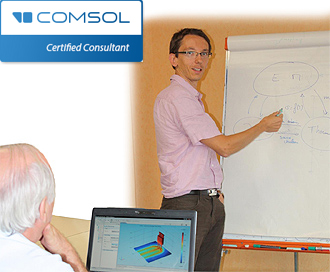Are you interested in learning :

• how to develop efficiently a model ?
• what are the main tips and tricks in modeling ?
• what are the common usual traps in modeling ?
• how to validate the numerical results ?
• how to acquire the theoretical background to develop our own model ?

Thanks to years of teaching experience, SIMTEC can build custom-made training sessions based on your needs. For clarity, each theoretical point is illustrated with several examples. Many exercises using the COMSOL Multiphysics software are developed to assure knowledge transmission.

Our training courses have been developed to make you independent in modeling. Here are some examples :

• Heat Transfer Modeling
• Transport Phenomena Modeling
• Electromagnetism Modeling
• Electrochemical phenomena Modeling
• CFD Modeling
• Basis of the Finite Element Method
• Mathematical Refresher on Partial Differential Equations (PDE)
• how to use efficiently COMSOL Multiphysics : mesh, solver, postprocessing, validation of modeling work.
• The easiest way to migrate from v3.5 to v4 of COMSOL Multiphysics™.

Simtec is approved as training institution.